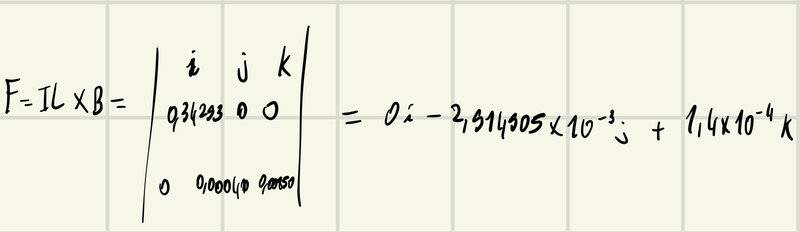# Magnetic force calculation

• kevinm829

#### kevinm829

Homework Statement
A wire 48.3 cm long carries a 0.710 A current in the positive direction of an x-axis through a magnetic field with an x component of zero, a y component of 0.000410 T, and a z component of 0.00850 T. Find the (a) x, (b) y, and (c) z components of the magnetic force on the wire.
Relevant Equations
Force on a wire: F=i*(crossP(L,B))
I am getting a wrong answer for part b, however part a and c both are correct :
a. 0 N
b = -2.91E-3 N (WRONG)
c. 1.4E-4 N

kuruman said: